Tangent at a Given Point on Other CurvesCustom SearchTANGENT AT A GIVEN POINT ON OTHER CURVES The technique used to find the slope and equation of the tangent line for a standard parabola can be used to find the slope and equation of the tangent line to a curve at any point regardless of the type of curve. The method can be used to find these relaŁtionships for circles, hyperbolas, ellipses, and general algebraic curves. This general method is outlined as follows: To find the slope, m, of a given curve at the point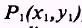, choose a second point, P', on the curve so that it has coordinates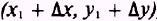; then substitute each of the coordinates of P' and P1 in the equation of the curve and simplify. Divide both sides byx and eliminate terms that contain powers ofy higher than the first power, as previously discussed. Solve for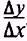. Letx approach zero andwill approach the slope of the tangent line, m, at point P1. When the slope and coordinates of a point on the curve are known, you can find the equation of the tangent line by using the point-slope method. EXAMPLE: Using the method outlined, find the slope and equation of the tangent line to the curve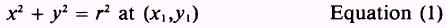SOLUTION: Choose a second point such that it has coordinatesSubstitute into equation (1)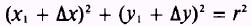ThusThenDivide both sides byx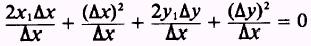and eliminating (y)2 results in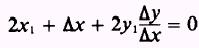Solve for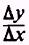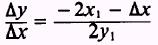Letx approach zero, so that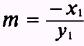Now using the point-slope form of a straight line, substitute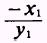for m: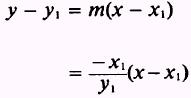Multiply both sides by y1Rearrange: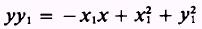but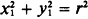Then, by substitution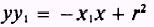andwhich is the general equation of the tangent line to the curveEXAMPLE: Using the given method, with minor changes, find the slope and equation of the tangent line to the curve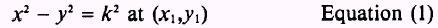SOLUTION: Choose a second point such that it has coordinatesSubstitute into equation (1):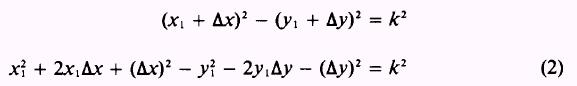Since, then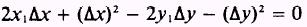Then divide byx and eliminate (y)2Solve for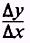Let Ax approach zero, so that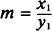which is the slope desired. Use the point-slope form of a straight line to find the equation of the tangent line to the curve at point (x1,y1) as shown in the following:Substitute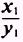for m: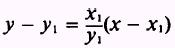Multiply both sides by y1: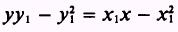Rearrange to obtain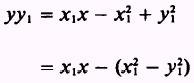Substitute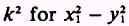;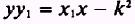Divide both sides by y1 to obtainwhich is the equation desired. PRACTICE PROBLEMS: Find the slope and equation of the tangent line to the curve, in problems 1 through 6, at the given points.ANSWERS: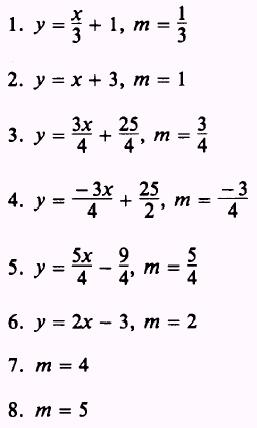Integrated Publishing, Inc. - A (SDVOSB) Service Disabled Veteran Owned Small Business